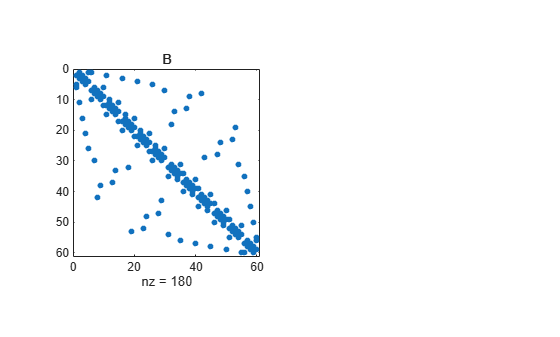# symrcm

## 语法

```r = symrcm(S) ```

## 说明

`r = symrcm(S)` 返回 `S` 的对称反向 Cuthill-McKee 排序。此置换向量 `r` 使得 `S(r,r)` 可能会使其非零元素更接近于对角线。对于源自长、瘦问题的矩阵的 LU 或 Cholesky 分解来说，这是一种很好的预排序方法。该排序方法对于对称和不对称 `S` 都有效。

## 示例

`B = bucky;`

```figure(); subplot(1,2,1),spy(B),title('B')``````p = symrcm(B); R = B(p,p);```

`spy` 图显示了更窄的带宽。

`subplot(1,2,2),spy(R),title('B(p,p)')``symamd` 的参考页中会继续对此示例进行说明。

```[i,j] = find(B); bw = max(i-j) + 1;```

`B``R` 的带宽分别为 35 和 12。

## 参考

 George, Alan and Joseph Liu, Computer Solution of Large Sparse Positive Definite Systems, Prentice-Hall, 1981.

 Gilbert, John R., Cleve Moler, and Robert Schreiber, “Sparse Matrices in MATLAB: Design and Implementation,” SIAM Journal on Matrix Analysis, 1992. A slightly expanded version is also available as a technical report from the Xerox Palo Alto Research Center.